# Here is a few nice things that Matlab user can do in Julia¶

array of ints

In :
x1 = [1:10]

Out:
10-element Array{Int32,1}:
1
2
3
4
5
6
7
8
9
10

array of floats

In :
x1 = [1.:10]

Out:
10-element Array{Float64,1}:
1.0
2.0
3.0
4.0
5.0
6.0
7.0
8.0
9.0
10.0

## Comprehensions¶

A = [ F(x,y,...) for x=rx, y=ry, ... ]
In :
[i+j for i=1:5, j=1:5]

Out:
5x5 Array{Int32,2}:
2  3  4  5   6
3  4  5  6   7
4  5  6  7   8
5  6  7  8   9
6  7  8  9  10
In :
x = rand(8)

Out:
8-element Array{Float64,1}:
0.604484
0.392061
0.159006
0.700496
0.016461
0.990571
0.56842
0.249582
In :
[ 0.25*x[i-1] + 0.5*x[i] + 0.25*x[i+1] for i=2:length(x)-1 ]

Out:
6-element Array{Any,1}:
0.386903
0.352642
0.394115
0.430997
0.641506
0.594249

## Plotting¶

In :
Pkg.add("Winston")

In :
using Winston

In :
plot(x1)

Out: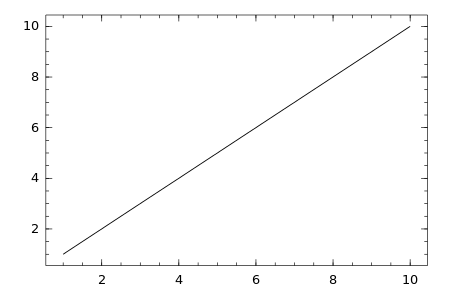In :
plot(x1, rand(10))

Out: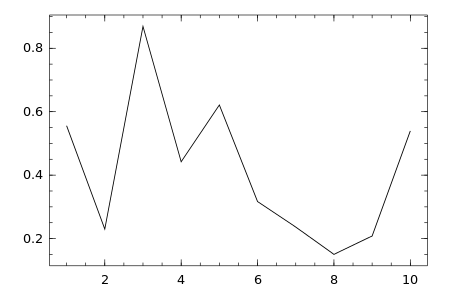In :
plot(x1, rand(10),"b-o")

Out: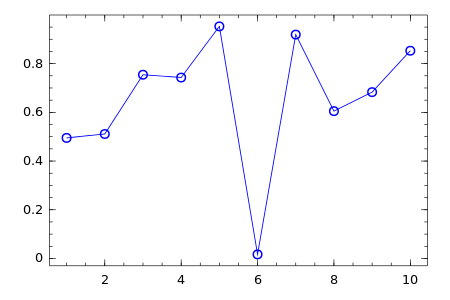In :
x2 = rand(10,10)

Out:
10x10 Array{Float64,2}:
0.470593   0.745078   0.00507957  0.55476    …  0.849684  0.61146   0.177914
0.0176863  0.854575   0.292973    0.416571      0.89902   0.336281  0.139129
0.559851   0.610792   0.42808     0.118449      0.267995  0.935479  0.234999
0.250439   0.0527366  0.838703    0.712658      0.561449  0.378895  0.317819
0.463184   0.629851   0.417069    0.544282      0.159377  0.844525  0.299531
0.873786   0.860217   0.0142058   0.236449   …  0.789597  0.668284  0.503
0.195273   0.179327   0.450923    0.14229       0.263772  0.132346  0.31263
0.89076    0.543465   0.0208126   0.922975      0.177622  0.988187  0.931534
0.236742   0.564753   0.299484    0.0745965     0.111413  0.209411  0.293134
0.608397   0.684601   0.846688    0.675908      0.613112  0.332819  0.422293
In :
imagesc(x2)
title("random matrix")

Out: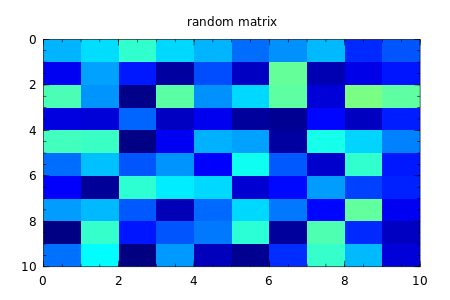## Nice way to express equations¶

In :
x = 5; y = 3;

In :
3x + 2y

Out:
21
In :
3x

Out:
15

### Unicode for everyone¶

In :
ρ(T,S) = ρ0*(1-α*(T-T0)+β*(S-S0))

Out:
ρ (generic function with 1 method)
In :
ρ0=1025
α = 2.5E-4
T0 = 20.
β = 8.E-4
S0 = 35.

Out:
35.0
In :
ρ(10,35)

Out:
1027.5625
In :
T = [10:15]

Out:
6-element Array{Int32,1}:
10
11
12
13
14
15
In :
S = [35.0:0.1:35.5]

Out:
6-element Array{Float64,1}:
35.0
35.1
35.2
35.3
35.4
35.5

In :
ρ(T,S)

Out:
6-element Array{Float64,1}:
1027.56
1027.39
1027.21
1027.04
1026.87
1026.69
In :
plot(ρ(T,S))

Out: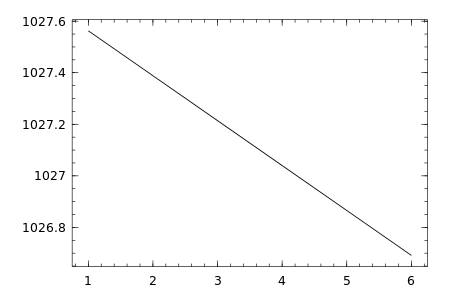## Strings¶

In :
st = "Hello there"

Out:
"Hello there"
In :
string(st[1:5]," ",st[7:9])

Out:
"Hello the"

## loops¶

In :
for letter in st
print(letter, "\n")
end

H
e
l
l
o

t
h
e
r
e

In :
for letter=st
print(letter, "\n")
end

H
e
l
l
o

t
h
e
r
e


## Evaluation of expressions inside strings¶

In :
"1 + 2 = $(1 + 2 + S)"  Out: "1 + 2 = 38.0" In : "First salinity is$(S) psu"

Out:
"First salinity is 35.0 psu"
In :
"All salinities are: \$S"

Out:
"All salinities are: [35.0,35.1,35.2,35.3,35.4,35.5]"

## Operators are functions¶

In :
+(1,2,3,4)

Out:
10
In :
*(1,2,3,4)

Out:
24

## Single expression which evaluates several subexpressions in order¶

In :
z = (x = 1+T; y = 2*S; x + y)

Out:
81.0

## a ? b : c¶

a ? b : c - The expression a, before the ?, is a condition expression, and the ternary operation evaluates the expression b, before the :, if the condition a is true or the expression c, after the :, if it is false.

In :
1 < 2 ? print("yes") : print("no")

yes
In :
danet(x) = (x>0) ? 1 : -1

Out:
danet (generic function with 1 method)
In :
danet(10)

Out:
1
In :
danet(-10)

Out:
-1

## Multiple nested for loops can be combined into a single outer loop, forming the cartesian product of its iterables:¶

In :
for i = 1:2, j = 3:4
println((i, j))
end

(1,3)
(1,4)
(2,3)
(2,4)


## System cals¶

In :
run(ls|grep U)

Untitled0.ipynb
Untitled1.ipynb